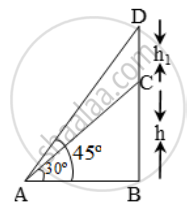# From a Point on the Ground 40 M Away from the Foot of a Tower, the Angle of Elevation of the Top of the Tower is 30º. the Angle of Elevatio - Mathematics

Sum

From a point on the ground 40 m away from the foot of a tower, the angle of elevation of the top of the tower is 30º. The angle of elevation of the top of a water tank (on the top of the tower) is 45º. Find the (i) height of the tower (ii) the depth of the tank.

#### Solution

Let BC be the tower of height h metre and CD be the water tank of height h1 metre.

Let A be a point on the ground at a distance of 40 m away from the foot B of the tower.In ∆ABD, we have tan 45º = \frac { BD }{ AB }

\Rightarrow 1=\frac{h+h_{1}}{40}\Rightarrow h+h_{1}=40\text{}m\text{ }\text{......}(i)

In ∆ABC, we have

\text{tan }30^\text{o}=\frac{BC}{AB}\Rightarrow\frac{1}{\sqrt{3}}=\frac{h}{40}

\Rightarrow h=\frac{40}{\sqrt{3}}=\frac{40\sqrt{3}}{3}=23.1\text{ m}

Substituting the value of h in (i), we get

23.1 + h1 = 40

⇒ h1 = (40 – 23.1)m = 16.9 m

Concept: Heights and Distances
Is there an error in this question or solution?